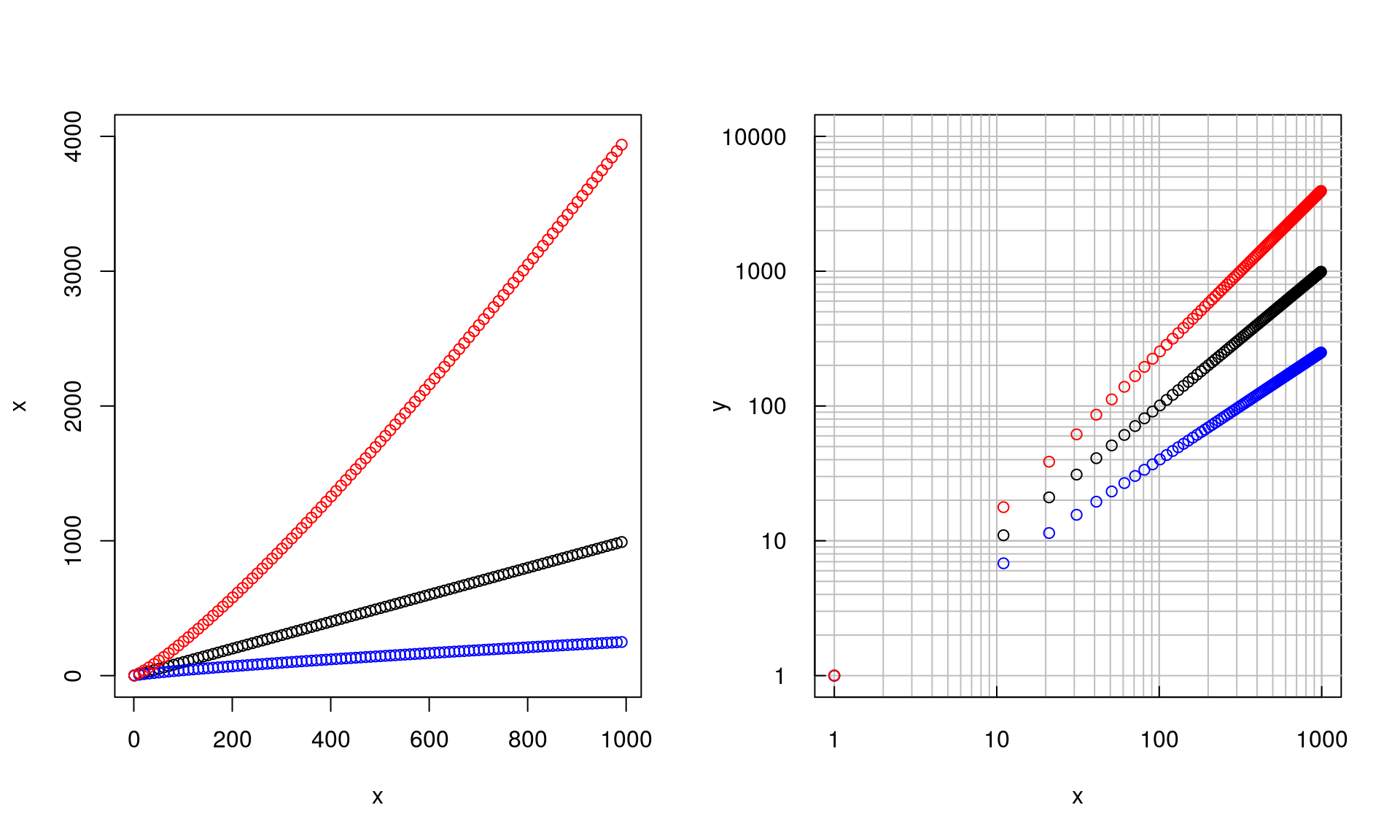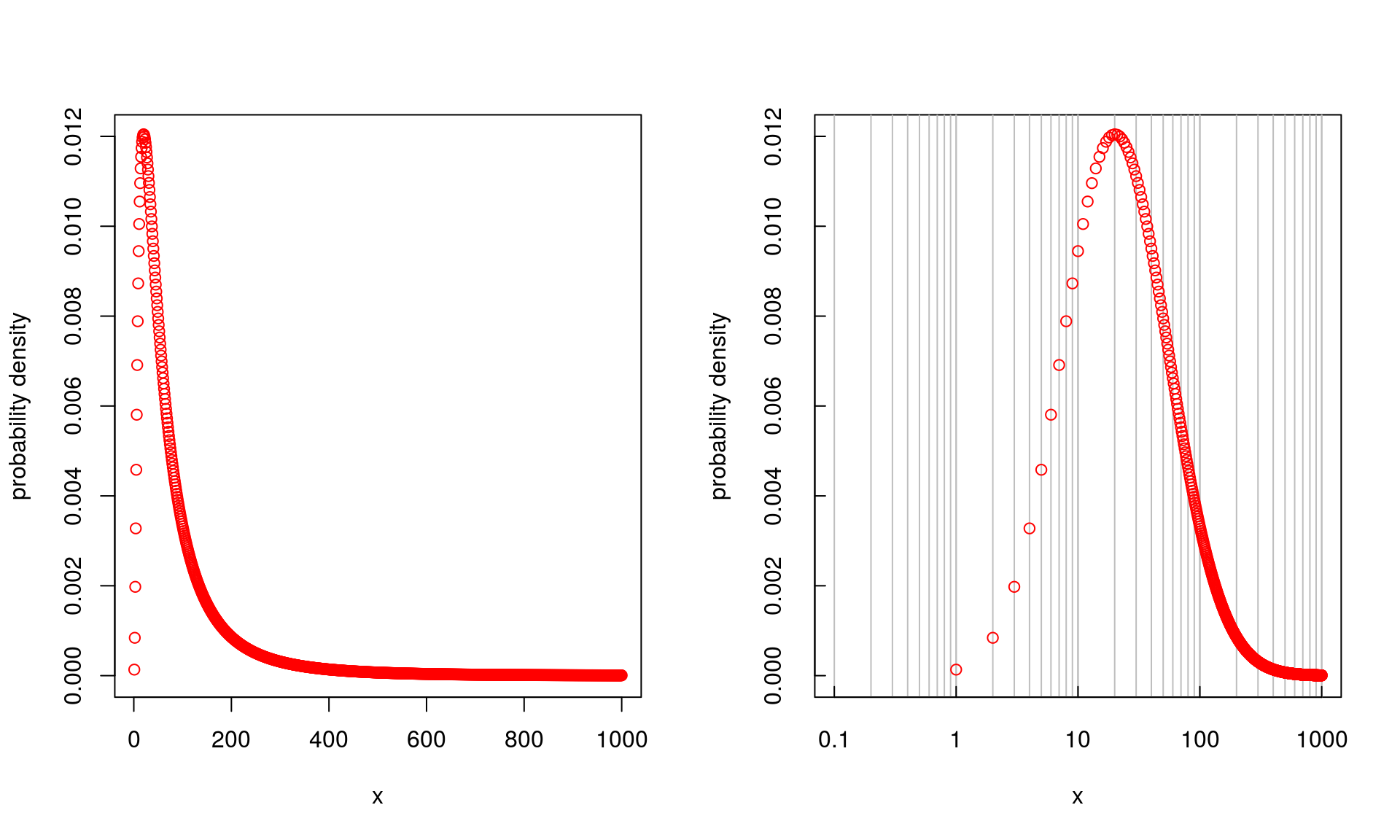Logarithmic axes with linear gridlines in basic R plots

By | July 18, 2016

I like Mathematica’s and Matlab’s log-log plots with logarithmic axes and linear tickmarks (and gridlines). In a way, they enable to imagine both multiplication and addition in a single figure. They also enable to more exactly visually connect data points with values.

I haven’t found a simple ‘one-liner’ that’d do such plots in R. In fact, I have always found R’s treatment of logarithmic axes a bit dull - I want the fancy gridlines!

Function loglog.plot

To provide the log-linear gridlines and tickmarks, I have wrtitten function loglog.plot.

To load the function from my GitHub repository:

source("https://raw.githubusercontent.com/petrkeil/Blog/master/2016_07_05_Log_scales/loglogplot.r")

Arguments:

xlim, ylim - Numeric vectors of length 2, giving the x and y coordinates ranges on linear scale.

xlog, ylog - Logical value indicating if x and y axes should be logarithmic (TRUE) or linear (FALSE). In case the linear scale is chosen, no gridlines are drawn.

xbase, ybase - Base of the logarithm of the respective axes. Ignored if linear axis is specified.

... - Further arguments to the generic R function plot.

Value:

Empty R base graphics plot, ready to be populated using lines, points and alike.

Example 1 - both axes logarithmic

Here I plot three power functions: one sub-linear (exponent = 0.8), one linear (exponent = 1) and one supra-linear (exponent = 1.2).

  par(mfrow=c(1,2))
x <- seq(1, 1000, by=10)

# left panel - both axes linear
plot(x, x, ylim=c(0,4000))
points(x, x^0.8, col="blue")
points(x, x^1.2, col="red")

# right panel - loglog plot
loglog.plot(xlab="x", ylab="y", ylim=c(1, 10000))
points(log10(x), log10(x))
points(log10(x), 0.8*log10(x), col="blue")
points(log10(x), 1.2*log10(x), col="red")Example 2 - x logarithmic, y linear

In this example I plot a lognormal probability density function, and I only plot the tickmarks and gridlines along the x-axis. The y-axis is linear.

  par(mfrow=c(1,2))
x <- 1:1000

# left panel - linear
plot(x, dlnorm(x, meanlog=4), ylim=c(0, 0.012),
col="red", ylab="probability density")

# right panel - loglog plot
loglog.plot(ylog=FALSE, ylim=c(0,0.012), xlim=c(0.1, 1000),
xlab="x", ylab="probability density", )
points(log10(x), dlnorm(x, meanlog=4), col="red")(Visited 4,555 times, 5 visits today)